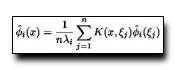Spectral Partitioning with Indefinite Kernels Using the Nyström Extension
Serge Belongie, Charless Fowlkes, Fan Chung, Jitendra MalikFowlkes et al. recently introduced an approximation to the Normalized Cut (NCut) grouping algorithm based on random subsampling and the Nyström extension. As presented, their method is restricted to the case where W, the weighted adjacency matrix, is positive definite. Although many common measures of image similarity (i.e. kernels) are positive definite, a popular example being Gaussian-weighted distance, there are important cases that are not. In this work, we present a modification to Nyström-NCut that does not require W to be positive definite. The modification only affects the orthogonalization step, and in doing so it necessitates one additional O(m^3) operation, where m is the number of random samples used in the approximation. As such it is of interest to know which kernels are positive definite and which are indefinite. In addressing this issue, we further develop connections between NCut and related methods in the kernel machines literature. We provide a proof that the Gaussian-weighted chi-squared kernel is positive definite, which has thus far only been conjectured. We also explore the performance of the approximation algorithm on a variety of grouping cues including contour, color and texture.

### Text Reference

Serge Belongie, Charless Fowlkes, Fan Chung, and Jitendra Malik. Spectral partitioning with indefinite kernels using the nyström extension. In ECCV, III: 531 ff. 2002.

### BibTeX Reference

@inproceedings{BelongieFCM_ECCV_2002,
AUTHOR = "Belongie, Serge and Fowlkes, Charless and Chung, Fan and Malik, Jitendra",
TITLE = {Spectral Partitioning with Indefinite Kernels Using the Nystr{\"o}m Extension},
BOOKTITLE = "ECCV",
YEAR = "2002",
PAGES = "III: 531 ff.",
TAG = "grouping"
}